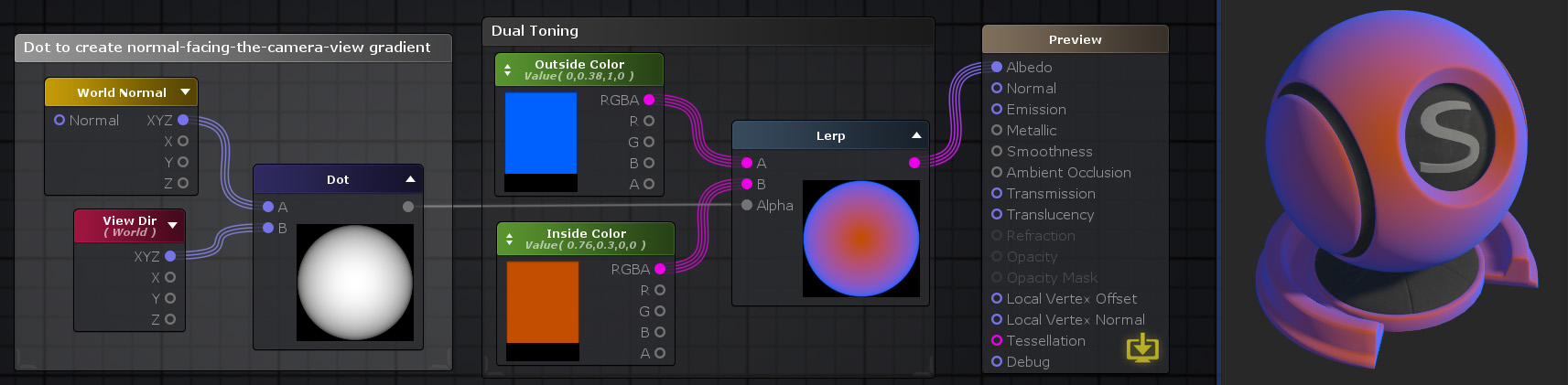# Unity Products:Amplify Shader Editor/Dot

Jump to: navigation, search

## Dot Node

The Dot node ( shortcut: Period key ) calculates a dot/inner product between two Vectors A and B. It geometrically represents the cosine of the angle formed between both vectors multiplied by each others lengths. In other words it outputs the sum of the multiplication of each vectors channels. P.e. if A and B are Vector3 then the result is A.x * B.x + A.y * B.y + A.z * B.z.

If A and B have different channel amounts a cast is done to match the one with the most amount of channels.

Note: Dot product is commutative so value's order is not important.Nodes used: Color, World Normal, View Dir, Dot, Lerp

Node Parameter Description Default Value
A First value of the dot product. Only visible if the respective input port is not connected. 0,0,0,0
B Last value of the dot product. Only visible if the respective input port is not connected. 0,0,0,0

Input Port Description Type
A First value of the dot product. Float4 
B Last value of the dot product. Float4 

1. ^ Port automatically adapts to all connection types except Matrices and Sampler types.